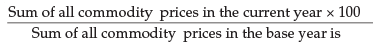Courses

# Test: Index Numbers- 1

## 40 Questions MCQ Test Quantitative Aptitude for CA CPT | Test: Index Numbers- 1

Description
This mock test of Test: Index Numbers- 1 for CA Foundation helps you for every CA Foundation entrance exam. This contains 40 Multiple Choice Questions for CA Foundation Test: Index Numbers- 1 (mcq) to study with solutions a complete question bank. The solved questions answers in this Test: Index Numbers- 1 quiz give you a good mix of easy questions and tough questions. CA Foundation students definitely take this Test: Index Numbers- 1 exercise for a better result in the exam. You can find other Test: Index Numbers- 1 extra questions, long questions & short questions for CA Foundation on EduRev as well by searching above.
QUESTION: 1

Solution:
QUESTION: 2

Solution:
QUESTION: 3

### _________ play a very important part in the construction of index nos.

Solution:
QUESTION: 4

________ is particularly suitable for the construction of index nos.

Solution:
QUESTION: 5

Index nos. show _________ changes rather than absolute amounts of change.

Solution:
QUESTION: 6

The ________ makes index nos. time-reversible.

Solution:
QUESTION: 7

Price relative is equal to

Solution:
QUESTION: 8

Index no. is equal to

Solution:
QUESTION: 9

The ________ of group indices given the General Index

Solution:
QUESTION: 10

Circular Test is one of the tests of

Solution:
QUESTION: 11

__________ is an extension of time reversal test

Solution:

Circular test Is an extension of time reversal test

QUESTION: 12

Weighted G.M. of relative formula satisfy ________test

Solution:
QUESTION: 13

Factor Reversal test is satisfied by

Solution:
QUESTION: 14

Laspeyres formula does not obey

Solution:
QUESTION: 15

A ratio or an average of ratios expressed as a percentage is called

Solution:
QUESTION: 16

The value at the base time period serves as the standard point of comparison

Solution:
QUESTION: 17

An index time series is a list of _______ nos. for two or more periods of time

Solution:
QUESTION: 18

Index nos. are often constructed from the

Solution:
QUESTION: 19

__________ is a point of reference in comparing various data describing individual behaviour.

Solution:
QUESTION: 20

The ratio of price of single commodity in a given period to its price in another period is called the

Solution:
QUESTION: 21Solution:
QUESTION: 22

Chain index is equal to

Solution:
QUESTION: 23

P01 is the index for time

Solution:
QUESTION: 24

P10 is the index for time

Solution:
QUESTION: 25

When the product of price index and the quantity index is equal to the corresponding value index then

Solution:
QUESTION: 26

The formula should be independent of the unit in which or for which price and quantities are quoted in

Solution:
QUESTION: 27

Laspeyres method and Paasche’s method do not satisfy

Solution:

Explanation : Unit test is a level of software testing. By this test, every unit of the software is tested. Laspeyres method and Paasche's method do not satisfy unit test

QUESTION: 28

The purpose determines the type of index no. to use

Solution:
QUESTION: 29

The index no. is a special type of average

Solution:
QUESTION: 30

The choice of suitable base period is at best temporary solution

Solution:
QUESTION: 31

Fisher’s Ideal Formula for calculating index nos. satisfies the _______ tests

Solution:
QUESTION: 32

Fisher’s Ideal Formula dose not satisfy _________ test

Solution:
QUESTION: 33

_________________ satisfies circular test

Solution:
QUESTION: 34

Laspeyre's and Paasche’s method _________ time reversal test

Solution:
QUESTION: 35

There is no such thing as unweighted index numbers

Solution:
QUESTION: 36

Theoretically, G.M. is the best average in the construction of index nos. but in practice, mostly the A.M. is used

Solution:
QUESTION: 37

Laspeyre’s or Paasche’s or the Fisher’s ideal index do not satisfy

Solution:
QUESTION: 38

___________ is concerned with the measurement of price changes over a period of years, when it is desirable to shift the base

Solution:
QUESTION: 39

The test of shifting the base is called

Solution:
QUESTION: 40

Which among the following statements is INCORRECT?

Solution: